# Exploratory data analysis in Chez Scheme

When I started learning Scheme, I took the common approach of learning a new language by implementing features from familiar languages (namely R). That sent me down the path of writing the chez-stats and dataframe libraries and porting gnuplot-pipe from Chicken to Chez Scheme. Those three libraries now allow me to conduct simple exploratory data analysis (EDA) in Chez Scheme that should be feel relatively familiar to R programmers. In this post, I will work through a simple example, which mostly serves to reinforce how much better suited R is for these types of tasks.

For this post, we will use the Texas housing data included as part of the ggplot2 package for R. I’ve written that dataset to a CSV file for use in this post. First, let’s import the necessary libraries (after following installation instructions in the repos linked above).

(import (chez-stats)
(dataframe)
(prefix (gnuplot-pipe) gp:))

chez-stats1 has a procedure for reading delimited text files, read-delim, where the default separator is a comma.

> (list-head (read-delim "txhousing.csv") 5)
(("city" "year" "month" "sales" "volume" "median" "listings" "inventory" "date")
("Abilene" "2000" "1" "72" "5380000" "71400" "701" "6.3" "2000")
("Abilene" "2000" "2" "98" "6505000" "58700" "746" "6.6" "2000.0833333333333")
("Abilene" "2000" "3" "130" "9285000" "58100" "784" "6.8" "2000.1666666666667")
("Abilene" "2000" "4" "98" "9730000" "68600" "785" "6.9" "2000.25"))

read-delim reads a CSV file as a list of lists with a row orientation. In the dataframe library, I refer to this type of list as a rowtable to distinguish it from the column-oriented dataframe, which is a record type based on an association list (alist).

The dataframe library includes pipeline operators taken from on an early implementation of SRFI 197.2 The -> places the output of the previous expression as the first argument of the next expression.

The #t in rowtable->dataframe indicates that the the rowtable has a header row. read-delim reads all data as strings. dataframe-modify-at allows us to map the same procedure, string->number, over several columns (e.g., year, sales, etc.).

> (define df1
(rowtable->dataframe #t)
(dataframe-modify-at
string->number 'year 'month 'sales 'volume 'median 'listings 'inventory 'date)))

> (dataframe-display df1)
city      year     month     sales    volume    median  listings  inventory
Abilene      2000         1        72   5380000     71400       701        6.3
Abilene      2000         2        98   6505000     58700       746        6.6
Abilene      2000         3       130   9285000     58100       784        6.8
Abilene      2000         4        98   9730000     68600       785        6.9
Abilene      2000         5       141  10590000     67300       794        6.8
Abilene      2000         6       156  13910000     66900       780        6.6
Abilene      2000         7       152  12635000     73500       742        6.2
Abilene      2000         8       131  10710000     75000       765        6.4
Abilene      2000         9       104   7615000     64500       771        6.5
Abilene      2000        10       101   7040000     59300       764        6.6
Columns not displayed: date

> (dataframe-dim df1)
(8602 . 9)

In Chez Scheme, there is no built-in representation for missing values (like NA in R). We have to handle them manually. In our example, we have already converted 8 of the 9 columns from strings to numbers and can use #f as an indicator of missing values. But this carries the limitation that typos (e.g., 701t) are treated as missing.3

> (map string->number '("one" "2" ""))
(#f 2 #f)

Here is a procedure for counting the number of #f values in each column of a dataframe. Because a dataframe record type is based on an alist, we can extract the alist from the dataframe with dataframe-alist and use standard Scheme procedures.

> (define (count-false df)
(map (lambda (col)
(length (filter (lambda (val) (not val)) (cdr col))))
(dataframe-alist df)))

> (count-false df1)
(0 0 0 568 568 616 1424 1467 0)

We need a dataframe with no missing values because we can’t pass missing values to the statistics and plotting procedures. Let’s drop the columns with the most missing values and then drop all rows with any #f values. The filter expression relies on the fact that all values (other than #f) count as true in a Scheme boolean expression.

>  (define df-complete
(-> df1
(dataframe-drop 'listings 'inventory)
(dataframe-filter-all (lambda (x) x))))

> (count-false df-complete)
(0 0 0 0 0 0 0)

> (dataframe-display df-complete)
city      year     month     sales    volume    median      date
Abilene      2000         1        72   5380000     71400      2000
Abilene      2000         2        98   6505000     58700  2000.083
Abilene      2000         3       130   9285000     58100  2000.166
Abilene      2000         4        98   9730000     68600   2000.25
Abilene      2000         5       141  10590000     67300  2000.333
Abilene      2000         6       156  13910000     66900  2000.416
Abilene      2000         7       152  12635000     73500    2000.5
Abilene      2000         8       131  10710000     75000  2000.583
Abilene      2000         9       104   7615000     64500  2000.666
Abilene      2000        10       101   7040000     59300   2000.75

Now that we have cleaned up the dataset we will aggregate the data for plotting to look for annual and seasonal patterns. dataframe-aggregate takes an aggregate-expr as one of its arguments. If we separately define an aggregate-expr, then we can easily use it with different grouping variables.

> (define agg-expr
(aggregate-expr
(avg-sales (sales) (exact->inexact (mean sales)))
(avg-volume (volume) (exact->inexact (mean volume)))
(avg-median (median) (exact->inexact (mean median)))))

> (define df-agg-year
(dataframe-aggregate df-complete '(year) agg-expr))

> (dataframe-display df-agg-year)
year  avg-sales  avg-volume  avg-median
2000  491.12444  73788386.9     96442.0
2001  510.40354  79052988.2  100801.552
2002  547.11709  88198732.5  104365.105
2003  540.12765  88653190.3  108030.638
2004  577.23373  97384530.2  111096.747
2005  635.90744  113752022.  118384.305
2006  664.88145  124737114.  124263.479
2007  620.64583  121958294.  130156.628
2008  520.29755  101945887.  131297.928
2009  470.64206  89349077.4  131483.763

We can plot data using gnuplot-pipe; a Chez Scheme interface for Gnuplot. I have only a rudimentary understanding of how to use Gnuplot so these plots will be very simple.

Below is an example of the gnuplot-pipe syntax for a simple line plot. The legend label is followed by lists for the x- and y-values.

(gp:call/gnuplot
(gp:plot "title 'x^2'" '(1 2 3 4) '(1 4 9 16))
(gp:save "OneLine.png"))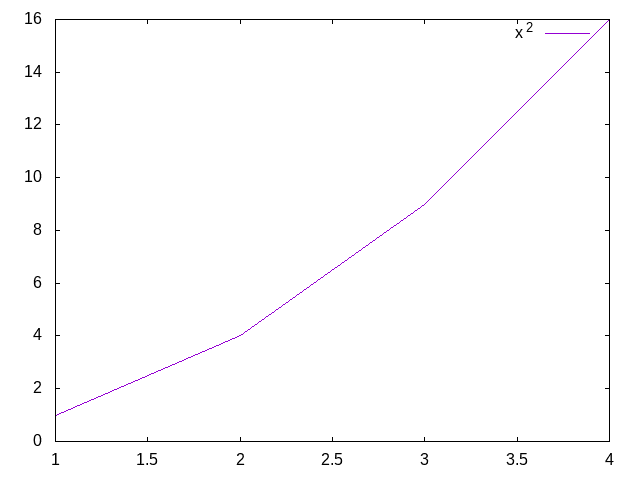When you want to plot multiple lines, you put the same syntax from the single line plot into a list with each element of the list representing a different line.

(gp:call/gnuplot
(gp:plot '(("title 'x^2'" (1 2 3 4) (1 4 9 16))
("title 'x^3'" (1 2 3 4) (1 8 27 64))))
(gp:save "TwoLines.png"))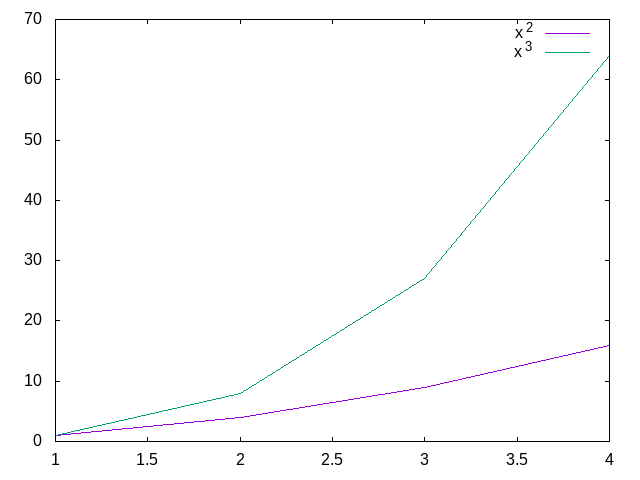The code for a plot with multiple lines based on a dataframe would be verbose and redundant. We can write a macro to simplify the plotting code. The plot-expr macro takes a dataframe as the 1st argument, x and y column names as the 2nd and 3rd arguments, and the grouping column name as an optional 4th argument. When a grouping argument is specified, plot-expr maps over the unique values in the grouping column to create the list structure expected by gp:plot.

(define-syntax plot-expr
(syntax-rules ()
[(_ df x y)
(list (list "" ($df (quote x)) ($ df (quote y))))]
[(_ df x y grp)
(map (lambda (grp-val)
(let ([df-sub (dataframe-filter df (filter-expr (grp) (string=? grp grp-val)))])
(list
(string-append "title '" grp-val "'")
($df-sub (quote x)) ($ df-sub (quote y)))))
(dataframe-values-unique df (quote grp)))]))

We can now use our plot-expr to plot the data that we aggregated by year across all cities and months. Here we are plotting the average median sale price by year. Nationally, home prices peaked in 2006 and bottomed out in 2012 (source), but, in Texas, housing prices mostly stayed flat during that period.

(gp:call/gnuplot
(gp:send "unset key")          ; remove legend
(gp:plot (plot-expr df-agg-year year avg-median))
(gp:save "MedianSalePriceByYear.png"))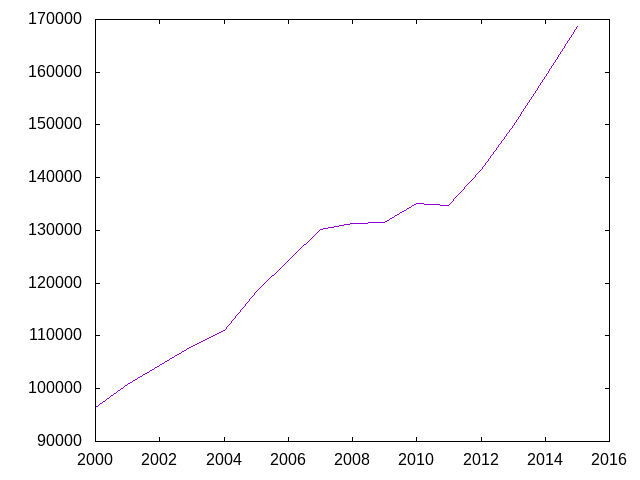Next, we create a filter-expr to filter by the largest cities in future aggregations.

(define city-filter
(filter-expr
(city)
(member city '("Austin" "Dallas" "El Paso" "Houston" "Lubbock" "San Antonio"))))

In this next code block, we filter for the selected cities, aggregate by city and year, and then bind the previously aggregated dataframe, df-agg-year, while adding a city column to df-agg-year with the value of All in every row.

(define df-agg-city-year
(->  df-complete
(dataframe-filter city-filter)
(dataframe-aggregate '(city year) agg-expr)
(dataframe-bind
(dataframe-modify
df-agg-year
(modify-expr (city () "All"))))))

The next plot includes 7 lines, but the plot-expr is mostly the same as for plotting one line.

(gp:call/gnuplot
(gp:send "set key top left")
(gp:plot (plot-expr df-agg-city-year year avg-median city))
(gp:save "MedianSalePriceByYearAndCity.png"))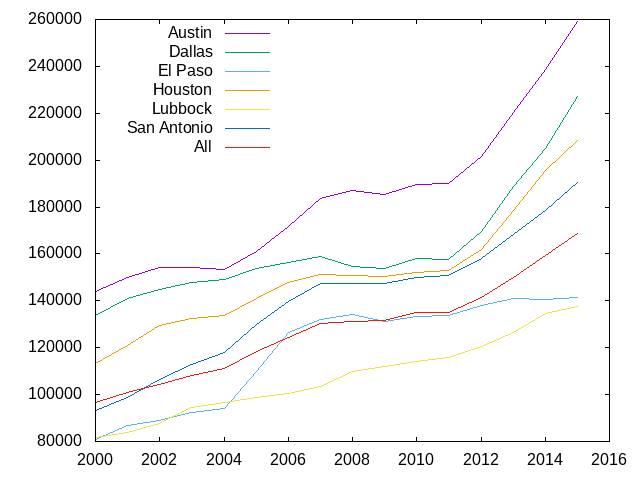The code is very similar for monthly patterns.

(define df-agg-month
(-> df-complete
(dataframe-aggregate '(month) agg-expr)))
(dataframe-sort (sort-expr (< month)))))

(define df-agg-city-month
(->  df-complete
(dataframe-filter city-filter)
(dataframe-aggregate '(city month) agg-expr)
(dataframe-sort (sort-expr (< month)))
(dataframe-bind
(dataframe-modify
df-agg-month
(modify-expr (city () "All"))))))

(gp:call/gnuplot
(gp:send "set key top left")
(gp:plot (plot-expr df-agg-city-month month avg-median city))
(gp:save "MedianSalePriceByMonthAndCity.png"))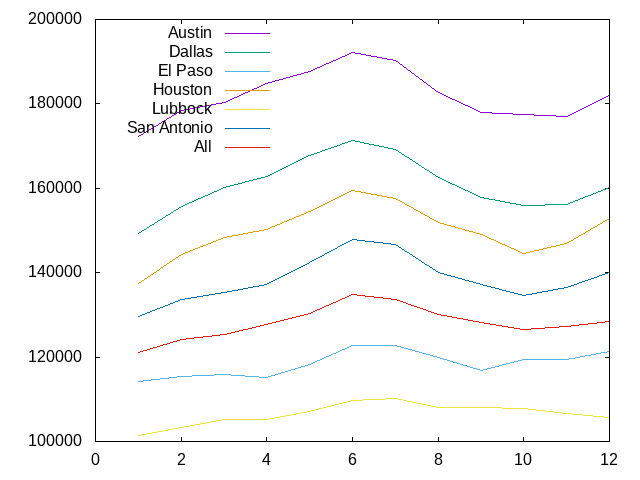In conclusion, it’s been fun to see that something I built is moderately useful for exploratory data analysis, but it would be a HUGE amount of work to take these libraries to a place where it could even partially replace R for me. To be clear, I wasn’t trying to replace R. I was just learning Scheme. I don’t anticipate investing too much more time in the dataframe or gnuplot-pipe libraries, but I might slowly add features to chez-stats.

1. In retrospect, read-delim is a better fit for the dataframe library.↩︎

2. SRFI 197 has now deviated considerably from that early syntax.↩︎

3. I’m not suggesting that this is an insurmountable problem, but it requires extra processing that is not done in this example.↩︎